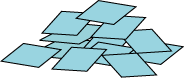#### You may also like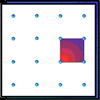### Geoboards

This practical challenge invites you to investigate the different squares you can make on a square geoboard or pegboard.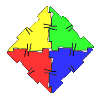### Polydron

This activity investigates how you might make squares and pentominoes from Polydron.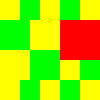### Tiles on a Patio

How many ways can you find of tiling the square patio, using square tiles of different sizes?

# A Square in a Circle

##### Age 7 to 11 Challenge Level:

Harry had a circle which was marked with twelve numbered dots to help him draw clock faces. The circle had a diameter of $10$ cm.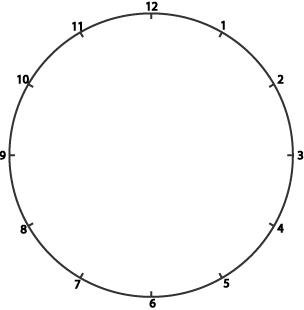Harry drew lines from the $12$ to the $3$, from the $3$ to the $6$, from the $6$ to the $9$, and then back from the $9$ to the $12$.